# Specific Resistance of Conductors

## Basic Electricity

• #### Question 1

Given two lengths of metal wire, which one will have the least electrical resistance: one that is short, or one that is long? Assume all other factors are equal (same metal type, same wire diameter, etc.).

• #### Question 2

Given two lengths of solid metal wire with round cross-sections, which one will have the least electrical resistance: one that is small-diameter, or one that is large-diameter? Assume all other factors are equal (same metal type, same wire length, etc.).

• #### Question 3

What is specific resistance, symbolized by the Greek letter “rho” (ρ)?

• #### Question 4

Write a single equation relating the resistance, specific resistance, length, and cross-sectional area of an electrical conductor together.

• #### Question 5

Examine the following specific resistance table for various metals:

 Metal type ρ in Ω · cmil / ft @ 32oF ρ in Ω · cmil / ft @ 75oF Zinc (very pure) 34.595 37.957 Tin (pure) 78.489 86.748 Copper (pure annealed) 9.390 10.351 Copper (hard-drawn) 9.810 10.745 Copper (annealed) 9.590 10.505 Platinum (pure) 65.670 71.418 Silver (pure annealed) 8.831 9.674 Nickel 74.128 85.138 Steel (wire) 81.179 90.150 Iron (approx. pure) 54.529 62.643 Gold (99.9 % pure) 13.216 14.404 Aluminum (99.5 % pure) 15.219 16.758

Of the metals shown, which is the best conductor of electricity? Which is the worst? What do you notice about the resistivity of these metals as temperature is increased from 32oF to 75 oF?

• #### Question 6

What is the electrical resistance of a 12-gauge copper wire, 500 feet long, at room temperature?

• #### Question 7

A spool holds an unknown length of aluminum wire. The size of the wire is 4 AWG. Fortunately, both ends of the wire are available for contact with an ohmmeter, to measure the resistance of the entire spool. When measured, the wire’s total resistance is 0.135 Ω. How much wire is on the spool (assuming the spool is at room temperature)?

• #### Question 8

The cross-sectional dimensions of a copper “busbar” measure 8 cm by 2.5 cm. How much resistance would this busbar have, measured end-to-end, if its length is 10 meters? Assume a temperature of 20o Celsius.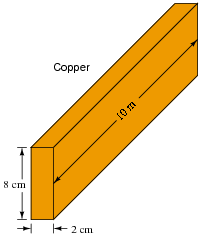• #### Question 9

Calculate the end-to-end resistance of a 20 meter length of copper wire with a diameter of 0.05 cm. Use 1.678 ×10−6 Ω·cm for the specific resistance of copper.

• #### Question 10

Calculate the amount of power delivered to the load resistor in this circuit: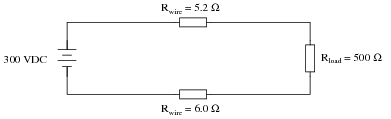Also, calculate the amount of power that would be delivered to the load resistor if the wires were superconducting (Rwire = 0.0 Ω).

• #### Question 11

Suppose a power system were delivering AC power to a resistive load drawing 150 amps:Calculate the load voltage, load power dissipation, the power dissipated by the wire resistance (Rwire), and the overall power efficiency, indicated by the Greek letter “eta” (η = [(Pload)/(Psource)]).

Plines =
η =

Now, suppose we were to re-design both the generator and the load to operate at 2400 volts instead of 240 volts. This ten-fold increase in voltage allows just one-tenth the current to convey the same amount of power. Rather than replace all the wire with different wire, we decide to use the exact same wire as before, having the exact same resistance (0.1 Ω per length) as before. Re-calculate load voltage, load power, wasted power, and overall efficiency of this (higher voltage) system: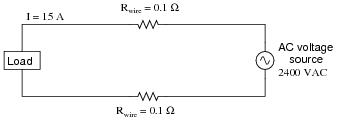Plines =
η =
• #### Question 12

The efficiency (η) of a simple power system with losses occurring over the wires is a function of circuit current, wire resistance, and total source power: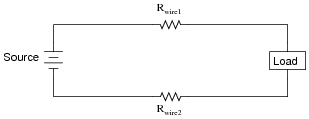A simple formula for calculating efficiency is given here:

 η = Psource − I2R Psource

Where,

Psource = the power output by the voltage source, in watts (W)

I = the circuit current, in amperes (A)

R = the total wire resistance (Rwire1 Rwire2), in ohms (Ω)

Algebraically manipulate this equation to solve for wire resistance (R) in terms of all the other variables, and then calculate the maximum amount of allowable wire resistance for a power system where a source outputting 200 kW operates at a circuit current of 48 amps, at a minimum efficiency of 90%.

• #### Question 13

What size (gauge) of copper wire is needed in this circuit to ensure the load receives at least 110 volts?• #### Question 14

A strain gauge is a type of sensing device widely used in the aerospace industry, for testing of vehicles and mechanical components. Explain what a strain gauge does, and how it functions.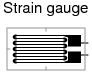• #### Question 15

How does the conductance (G) of a conductor relate to its length? In other words, the longer the conductor, the (fill-in-the-blank

) its conductance is, all other factors being equal.